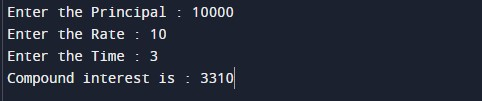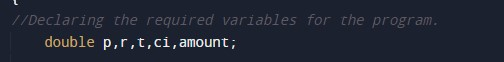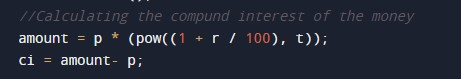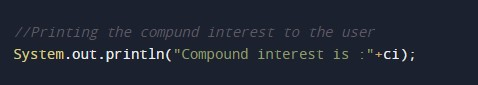# Java Program to Calculate Compound Interest

In this tutorial you will learn about the Java Program to Calculate Compound Interest and its application with practical example.

## Java Program to Calculate Compound Interest

In this tutorial, we will learn to create a Java program that will calculate compound interest using Java programming.

## Prerequisites

Before starting with this tutorial, we assume that you are the best aware of the following Java programming topics:

• Operators in Java Programming.
• Basic Input and Output function in Java Programming.
• Basic Java programming.
• Conditional Statements in Java programming.
• Arithmetic operations in Java Programming.

## What Is Compound Interest?

Compound Interest is an interest that obtains by adding the interest of each year to the principal sum and then calculating the interest on the updated amount.

CI is interest earned from the original principal +  accumulated interest.

## Formula for Compound Interest is.Here,
is principle amount.
is the rate in Percent %.
is time in year.

## Program Description:-

In this program, First, we will take the rate, time, and the principal amount from the user. Then we will calculate the compound interest of that amount for the time using the mathematical expression. At last, We will print the compound interest from the program.

Below is an example program.

## OutputIn the above program, we have first initialized the required variable.• p = it will hold the double principal value from the user.
• r = it will hold the double rate value from the user.
• t = it will hold the double-time value from the user.
• amount  = it will hold the ci value.
• ci  = it will hold the double value.

Calculating the Compound interest of the program.Printing the output to the user.In this tutorial we have learn about the Java Program to Calculate Compound Interest and its application with practical example. I hope you will like this tutorial.